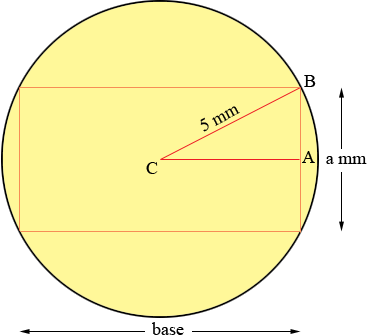SEARCH HOMEMath Central Quandaries & QueriesFelicia, a parent: A rectangle whose base is twice its altitude is inscribed in a circle whose radius is 5 mm. Find the area of the rectangle.Hi Felica,
In my diagram I let a mm be the altitude of the rectangle. What is the length of the base?Angle CAB is a right angle. What is the length of AB? What is the length of CA? What does Pythagoras theorem say for triangle CAB?

Solve for a.

PennyMath Central is supported by the University of Regina and The Pacific Institute for the Mathematical Sciences.